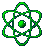Deutsch
6thfloor.de » Science Net    Home > The number 666Categories
Other
InteractiveVisitors since 3/2000

Last Update:
09.03.2002

# Little pastime with figures

Here something for the numerary mythologists and the ones who saw the film “End of days” (by the way a very good film!) and all who are interested. The number 666, the figure of devil.
This figure comes from a manifestation of Johannes in that a brute with the designation 666 is described.
What exactly is meant is not important for this. More interesting is the way to find this figure.
I listed some examples here, surely there will be some other but I`m just having material for only them. Here a well known example: If you write the name of the roman emperor Nero in hebrew so the worth of the figures would come to 666.
Some other surprising possibilities that we can prove:

“LATINUS REX SACERDOS” and ”VICARIUS FILII DEI”

latinus = latin rex = king, sovereign

sacerdos = priest vicarius = vicarious

filii = son (from filius) dei = god

The one phrase you can find on the Tiara, the crown of the pope, the other above the entrance of the Vatican.

If you look at this letters with care you can see roman figures.

The roman figures:

I = 1   V = 5   X = 10   L = 50

C = 100   D = 500   M = 1000

Let's have a look on the first phrase:

L, I, V, X, C, D.

The V is the same as an U, cause there is only one symbol for both in latin.

So lets summarize this numbers:

50+1+5+10+100+500 = 666

The second phrase:

V, I, C, I, V, I, L, I, I, D, I

Summarize:

5+1+100+1+5+1+50+1+1+500+1 = 666

Another interesting example:

If you code the alphabet like this

A=100 B=101 C=102 etc.

and now put in the name "Hitler", you get:

107+108+119+111+104+117 = 666

Coincidence?

Written by [Andi]